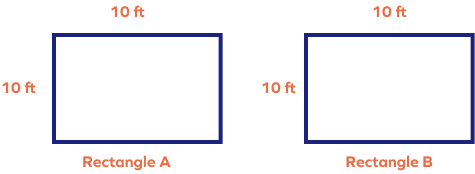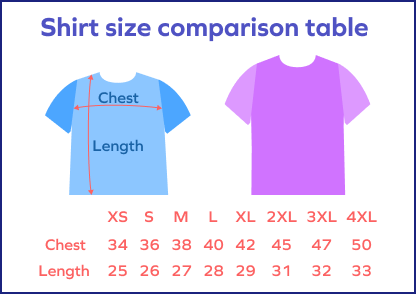# Size – Definition with Examples

## What Is Size?

Size in math is a relative concept. We always talk about the size of one thing in comparison with another thing. We use it to tell how small or big an object is or to measure things and determine their dimensions. In simple words, size refers to the overall measurement and dimensions of something.

The mathematical dimension of an object remains the same, but the size of an object may differ depending on various factors like who is seeing it, how far you are viewing it from, etc. For instance, a dog might seem small to an adult, whereas the same dog can look very big to a toddler.

## Size Definition

Size can be defined as the measurement of an object or a thing using its overall dimensions. The size tells us how big or small something is.

We talk about the size of different shapes depending on different dimensions, such as length, volume, mass. We will discuss a few examples in the next section!

## Size of Objects

We come across many instances where we make decisions based on the “size” of the object. Since it is a relative concept, we use the words like “bigger,” “smaller,” “greater,” etc., to make sense of the comparison. Let’s look at some examples in daily life.

Example 1:

Suppose you wish to buy a new T-shirt. There are different types of sizes available in the shops: “XS,” “S,” “M,” “L,” “XL,” “XXL,” etc. How to check if a particular size will fit us or not? We can see the size guide or size chart. It mentions how different sizes fit for different body dimensions. So, you have to check if your body measurement matches the measurements given on the particular size.

Example 2:

The coffee comes in different size containers. The size is decided by the quantity of coffee (volume) filled in the cup. The prices are also set accordingly.

## Measurement of Objects

As we discussed earlier, the size refers to the dimensions of an object, i.e., width, length, and height. Sometimes it may refer to the mass or weight or volume of an object. It is important to keep in mind that the size of an object is measured depending on its shape. For instance, a rectangle will be measured differently from a circle.

If a child wears his father’s shoes, it won’t fit! However, if we properly measure the feet size and match it with the shoe size, we will get a perfect fit. So, measurements are important.

Let’s see a few examples of how we generally compare sizes of different shapes.

Example 1: Size of a circle depends on its radius. The following image shows circles of different sizes drawn using different radii.

Example 2: The following image shows dimensions of a cuboid, which determines its size.

Example 3: To determine which line segment is longer, we measure the individual lengths. The line that is longer in length will also be bigger in size.

Line B is longer. So, it is also bigger in size.

Example 4: The size of a square depends on the length of its side. A square with bigger side length will be bigger in area.

Example 4: A rectangle has two dimensions: length and width. So, its size depends on both these dimensions.

The perimeter of a rectangle is the total length of its boundary.

P $=$ length $+$ length $+$ width $+$ width

Area is the space bounded by its perimeter.

Area $=$ length $\times$ width

Example 5: Area of a triangle depends on the length of its sides and internal angles. It helps to determine the size of the triangle.

Area of a triangle $= \frac{1}{2} \times$ base $\times$ height

## Fun Facts!

1. Both human feet are rarely of the same size. The left foot is generally a few inches bigger in size compared to the right foot.
1. The smallest bones in the human body are found in the ears.
1. The Sun is enormous compared to the Earth. The diameter of the sun is about 109 times greater than the diameter of the Earth.

## Conclusion

In this article, we learnt that “size” is a relative concept and explored some real life examples as well. Let’s solve a few problems for better understanding.

## Solved Examples

1. How is the size of a circle determined?

Solution:

The size of the circle is determined by calculating the radius, which is the distance between the center and the circle’s border. The circle with greater radius will have greater area and thereby will be greater in size.

2. A blue square cardboard has 5 feet side length and a yellow square cardboard is 8 feet side length. Which cardboard is bigger in size?

Solution:

The size of the square is determined by its side length. Since the side of the yellow cardboard is greater than the blue one, the yellow cardboard is bigger in size.

3. Brian has a pile of newspapers that weighs 45 pounds, whereas Justin’s pile weighs 43.5 pounds. Whose pile will be bigger in size?

Solution:

Brian’s pile is bigger in size because his pile weighs more than Justin’s.

5. Which circle is bigger in size? Why?

Solution:

The first circle is bigger than the second circle, since its radius is greater.

## Practice Problems

1

### A ribbon is 45 ft long. If we cut 32 ft from it; how long is the ribbon now?

13 ft
12 ft
11 ft
10 ft
CorrectIncorrect
$45 - 32 = 13$ feet
2

### Two rolls of tape are 40 ft and 36 ft long. What is the total length if we join together to make one big roll?

72 ft
56 ft
86 ft
76 ft
CorrectIncorrect
$40 + 36 = 76$ feet
3

### Rectangle A is ____ compared to rectangle B.bigger
smaller
the same size
wider than
CorrectIncorrect
Correct answer is: the same size
Both the rectangles have the same dimensions. So, they are of the same size.
4

### Ron wants to buy a T-shirt. Which size should he buy if his chest measurement is 36 inches? Use the size chart.XS
S
XL
L
CorrectIncorrect
According to the size chart, a person with 36 inches chest size should buy a small sized T-shirt.

L $\times$ W $\times$ H refers to the three dimensions of a solid object: length, width, height.

Shape describes the appearance or the form of an object. Size describes the dimensions.

Example: Suppose a box has the shape of a cube. Its size is determined by its dimensions like length, breadth, height.

Length denotes how long the shape is, whereas height indicates how tall the shape is.

TV screen size is determined diagonally, from one corner to the opposite corner.

Depth is measured from top to bottom. Height is measured from the bottom to the top, or from the ground level upwards.